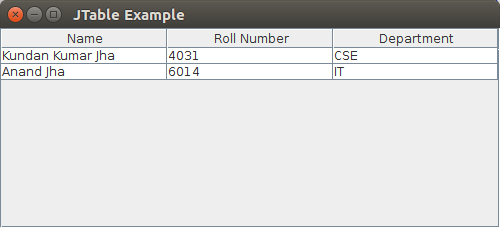# Java Swing | JTable

• Difficulty Level : Medium
• Last Updated : 12 Oct, 2021

The JTable class is a part of Java Swing Package and is generally used to display or edit two-dimensional data that is having both rows and columns. It is similar to a spreadsheet. This arranges data in a tabular form.
Constructors in JTable

1. JTable(): A table is created with empty cells.
2. JTable(int rows, int cols): Creates a table of size rows * cols.
3. JTable(Object[][] data, Object []Column): A table is created with the specified name where []Column defines the column names.

Functions in JTable

1. addColumn(TableColumn []column) : adds a column at the end of the JTable.
2. clearSelection() : Selects all the selected rows and columns.
3. editCellAt(int row, int col) : edits the intersecting cell of the column number col and row number row programmatically, if the given indices are valid and the corresponding cell is editable.
4. setValueAt(Object value, int row, int col) : Sets the cell value as ‘value’ for the position row, col in the JTable.

Below is the program to illustrate the various methods of JTable:

## Java

 `// Packages to import``import` `javax.swing.JFrame;``import` `javax.swing.JScrollPane;``import` `javax.swing.JTable;` `public` `class` `JTableExamples {``    ``// frame``    ``JFrame f;``    ``// Table``    ``JTable j;` `    ``// Constructor``    ``JTableExamples()``    ``{``        ``// Frame initialization``        ``f = ``new` `JFrame();` `        ``// Frame Title``        ``f.setTitle(``"JTable Example"``);` `        ``// Data to be displayed in the JTable``        ``String[][] data = {``            ``{ ``"Kundan Kumar Jha"``, ``"4031"``, ``"CSE"` `},``            ``{ ``"Anand Jha"``, ``"6014"``, ``"IT"` `}``        ``};` `        ``// Column Names``        ``String[] columnNames = { ``"Name"``, ``"Roll Number"``, ``"Department"` `};` `        ``// Initializing the JTable``        ``j = ``new` `JTable(data, columnNames);``        ``j.setBounds(``30``, ``40``, ``200``, ``300``);` `        ``// adding it to JScrollPane``        ``JScrollPane sp = ``new` `JScrollPane(j);``        ``f.add(sp);``        ``// Frame Size``        ``f.setSize(``500``, ``200``);``        ``// Frame Visible = true``        ``f.setVisible(``true``);``    ``}` `    ``// Driver  method``    ``public` `static` `void` `main(String[] args)``    ``{``        ``new` `JTableExamples();``    ``}``}`

OutputMy Personal Notes arrow_drop_up